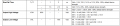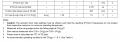Rise time vs Slew Rate - Trying to see if this circuit will work

matthej

Joined Oct 10, 2020
59
Hi,

I am trying to drive a 100MHZ clock oscillator to a LS1046 processor. The specifications are as follows:

100MHz clock:

The LS1046 processor :

The processor list slew rate as 1V/ns to 4V/ns. It is measured over a region of .35XOVDD to .65XOVDD where OVDD is 1.8V So over the range of .63V to 1.17V which is .54V

So the rise time for 1V/ns is .54/1 = .54ns and for 4V/ns is .54/4 = .135ns

So the Oscillator has a rise/fall time of 2.5ns at 20% to 80%, the Voh = 90% of VDD and Vol = 10% of Vdd. For Vdd = 1.8, Voh = 1.62 and Vol=.18. So 20% of that is .288V and 80% of that is 1.15V. So 1.15-.288 =.862V over 2.5ns which comes out to .344V/ns which looks too slow for the input clock.Does this make sense?

nsaspook

Joined Aug 27, 2009
9,088